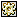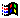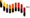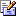Contents Articles Behaviors Books Director News Director Web Sites FAQ Games Mailing Lists News Groups Project Examples Reviews Software Tools Useful Web Sites Utilities Xtras

 Don't miss these#Compress integer list

 Compatibilities:This item has not yet been rated

Author: BrentMoseley

converts a list like this: [1, 1, 1, 1, 4, 4, 4, 1, 2] to this ["4 1", "3 4", 1, 2] or vice-versa, all without using globals. useful if you want to send a lot of integers across a mulit-user connection. to compress into a new list: "newlist = complist(somelist)" to decompress into a new list: "newlist = decomplist(compressedlist)"

 --Created by Brent Moseley  brentmoseley@yahoo.com --8/8/2000 --to compress into a new list:   "newlist = complist(somelist)" --to decompress into a new list: "newlist = decomplist(compressedlist)" on complist a, b, c, d   if b = void then b = 1   if c = void then c = 1   if d = void then d = []   --^sets up the variables on first time run.   --reference:   --a: orig list to pull from.   --b: number of redundant items in a row.   --c: current item number it"s checking.   --d: compressed output list.   repeat with x = 1 to count(a)     if the ilk of a[x] <> #integer then       alert "ERROR" & return & "Cannot compress non-integers"       return     end if   end repeat   --^makes sure any non-integers don"t slip through.   if c < count(a) then  --checks if it"s through the list yet.     --if two items in a row are the same it adds to the redundant count.     if a[c] = a[c+1] then       b = b + 1     else       --if the redudnant count is 3 or more it adds the compressed value to the final list.       if b > 2 then         add d, string(b) && a[c]       else if b > 1 then         add d, a[c]         add d, a[c]       else         add d, a[c]       end if       b = 1--reset the redundant count.     end if     c = c + 1     return complist(a,b,c,d)     --^moves on to the next item and re-runs the function.   else  --if it"s done running through the list add any remaining items and return final list.     if b > 1 then       add d, string(b) && a[c]     else       add d, a[c]     end if     return d   end if end on decomplist a   mydecomplist=[]   repeat with x = 1 to count(a)     --if the current item is a compressed string then decompress it and add it to the list.     if the ilk of a[x] = #string then       repeat with y = 1 to the value of word 1 of a[x]         add mydecomplist, the value of word 2 of a[x]       end repeat     else       add mydecomplist, a[x]     end if   end repeat   return mydecomplist end

 Contact MMI 36 South Court Sq Suite 300 Newnan, GA 30263 USASend e-mail